# plzzzz.... explain meaning of conservative force feilds with an example

11 years ago

conservative force is the force, work done by which is independent of the path followed but depends just on the initial and the final points.

Example is gravitational force.

Explanation: suppose a body reaches a height H by first stairs, then by lift, and finally via an inclined plane. In all the cases net displacement is the same, <mind it not the distance>

So the magnitude of work done will also be the same which is weight of the body*displacement.

So the work done against gravitational force will be same.

DO APPROVE IF YOU LIKE

DEEKSHA

.

.

.AKASH GOYAL AskiitiansExpert-IITD
419 Points
11 years ago

Dear Kedar

A conservative force is a force with the property that the work done in moving a particle between two points is independent of the path taken.   Equivalently, if a particle travels in a closed loop, the net work done  by a conservative force is zero

Suppose that a non-uniform force-field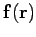acts upon an object which moves along a curved trajectory, labeled path 1, from pointto point. See Fig.. the work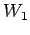performed by the force-field on the object can be written as a line-integral along this trajectory: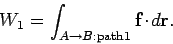(1)

Suppose that the same object moves along a different trajectory, labeled path 2, between the same two points. In this case, the work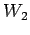performed by the force-field is(2)

Basically, there are two possibilities. Firstly, the line-integrals (1) and (2) might depend on the end points,and, but not on the path taken between them, in which case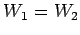. Secondly, the line-integrals (1) and (2) might depend both on the end points,and, and the path taken between them, in which case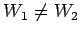(in general). The first possibility corresponds to what physicists term a conservative force-field, whereas the second possibility corresponds to a non-conservative force-field.Informally, a conservative force can be thought of as a force that conserves mechanical energy The gravitational force, spring force, magnetic force (according to some definitions, see below) and electric force (at least in a time-independent magnetic field, see Faraday's law of induction for details) are examples of conservative forces, while friction and air drag are classical examples of non-conservative forces (in both cases, the energy is converted to heat and cannot be retrieved)   All the best. AKASH GOYAL AskiitiansExpert-IITD   Please feel free to post as many doubts on our discussion forum as you can. We are all IITians and here to help you in your IIT JEE preparation. Win exciting gifts by answering the questions on Discussion Forum. So help discuss any query on askiitians forum and become an Elite Expert League askiitian. Now you score 5+15 POINTS by uploading your Pic and Downloading the Askiitians Toolbar  respectively : Click here to download the toolbar..